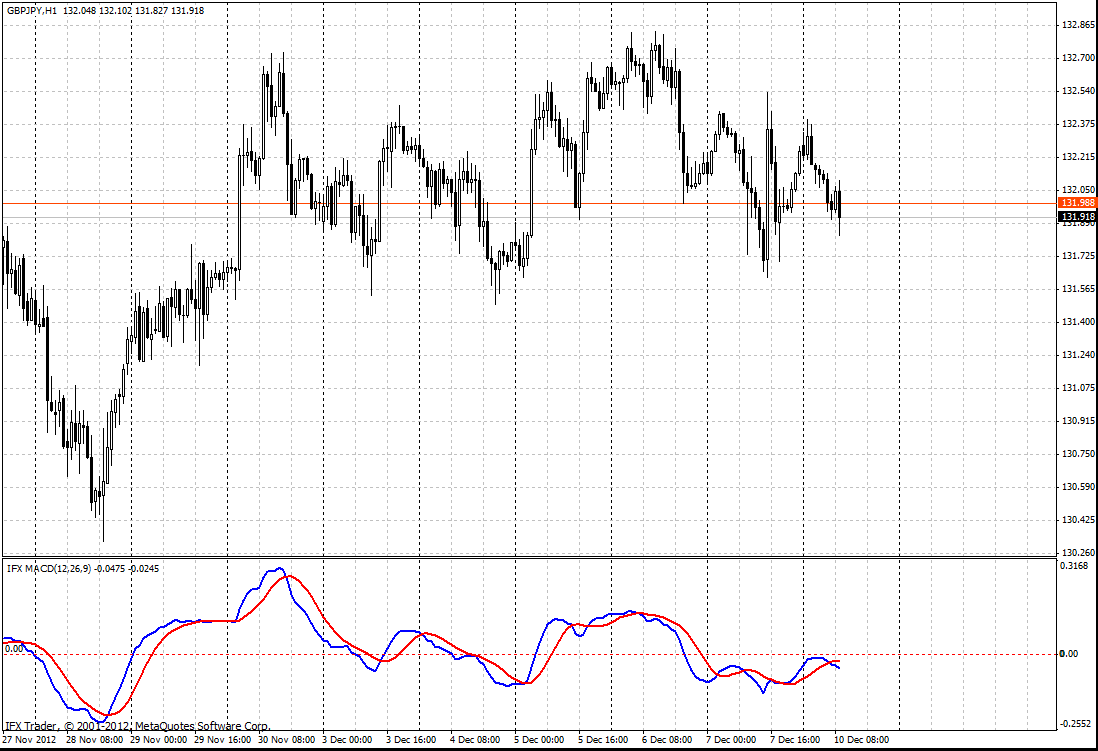#### 公式

MACD = (ЕМАs(P) – EMAl(P)),

Signal = SМАa(ЕМАs(P) – EMAl(P)), where

ЕМАl(P) is the exponential moving average with long period from price;

EMAs(P) is the exponential moving average with short period from price;

SМАa(P) is the smoothed moving average with a short period from the difference of the rest moving averages;

P – 收盘价。

#### 交易使用

MACD是一组振荡器中的一个经典的市场振荡器同样执行平面运动和运动趋势。与随机的振荡器只应用于价格在横向区间运行相比，MACD拥有更多功能。

MACD指标标准（未优化）设置最合适的时间帧是H4，每日，每周。FastEMA = 12

SlowEMA = 26

SignalSMA = 9